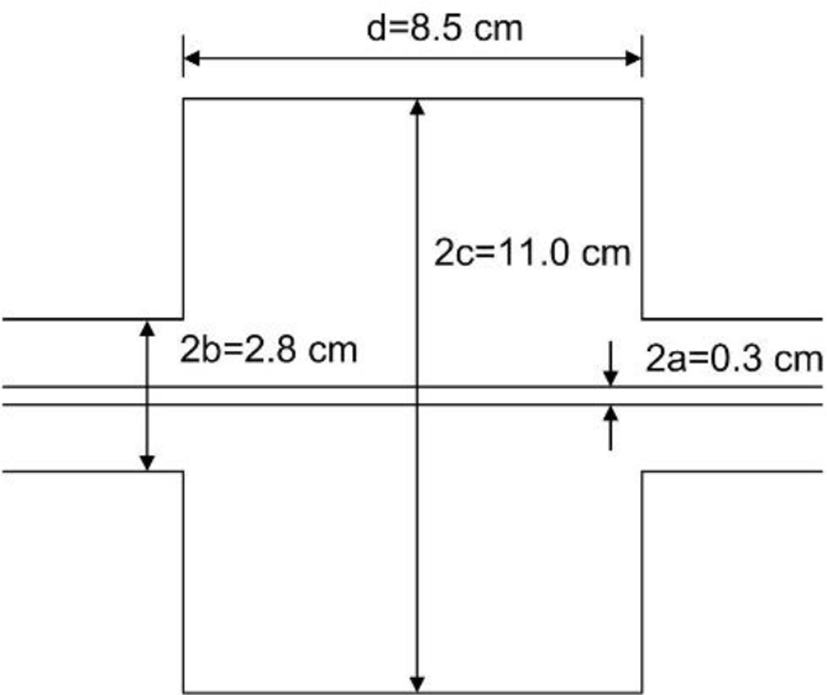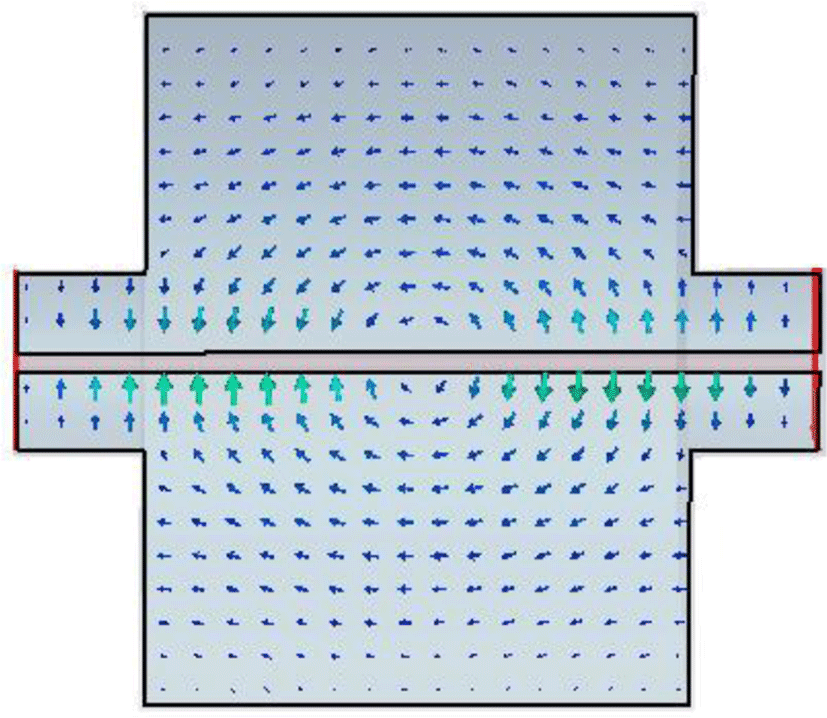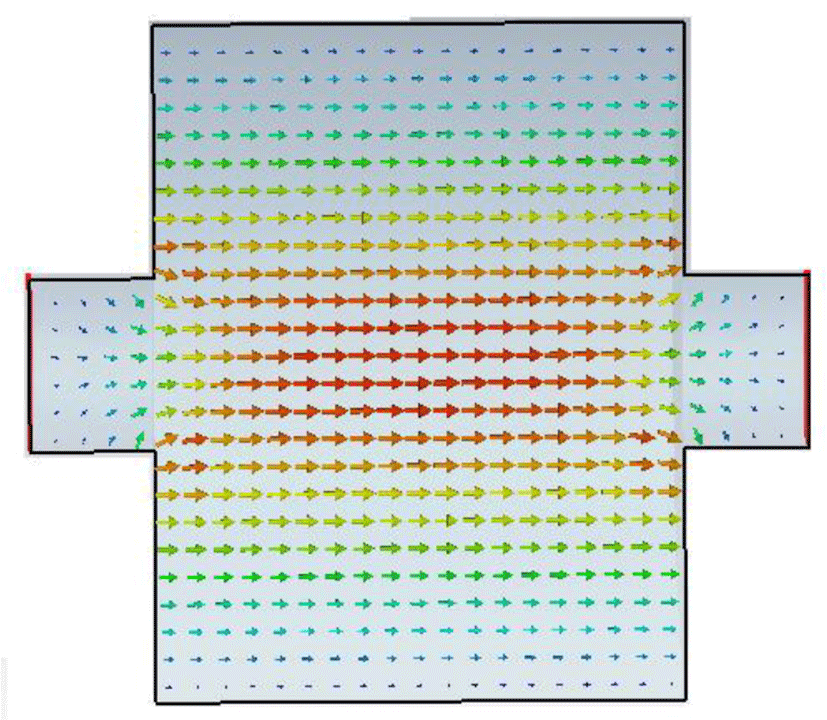Section D

# Brief Paper: Mode Matching Technique in a Cylindrical Cavity with Center Wire

Dae Hyun Han *
*Corresponding Author: Dae Hyun Han, Dept. of Electronic Engineering, Dongeui University, 176 Eomgwang-ro, Busanjin-gu, Busan, 47340, Republic of Korea, +82-51-890-1681, dhan@deu.ac.kr

© Copyright 2018 Korea Multimedia Society. This is an Open-Access article distributed under the terms of the Creative Commons Attribution Non-Commercial License (http://creativecommons.org/licenses/by-nc/4.0/) which permits unrestricted non-commercial use, distribution, and reproduction in any medium, provided the original work is properly cited.

Received: May 17, 2018 ; Revised: Jun 11, 2018 ; Accepted: Jun 21, 2018

Published Online: Jun 30, 2018

## Abstract

The eigen value problem of a coaxial cavity and a modified pill box cavity is investigated using the mode matching technique. The coaxial cavity has a cylindrical cavity with beam ports and center conductor. The pill box cavity is the same as a coaxial cavity without center conductor. The electric field and magnetic field are formulated in propagation region and resonance region. The boundary and orthogonal conditions are applied to the electric and magnetic fields. We derived the eigen value equation by the proposed procedure in a coaxial cavity and a modified pill box cavity. The electromagnetic field of the real structure is disturbed by the coaxial wire. The effect of the coaxial wire in pill box cavity with beam ports increase the dominant resonant frequency. The coaxial line method of the coupling impedance is not adequate for a cylindrical cavity. The results of the mode matching technique and simulation agree well. The results confirm the proposed formulation is valid.

Keywords: Coaxial cavity; Mode matching technique; Numerical simulation; Pill box cavity

## I. INTRODUCTION

Mode matching technique is one of the most frequently used methods for formulating boundary values problem. The mode matching method has been described in detail by Itoh . Mode matching technique has been applied to various fields such as the analysis of metal strip loaded dielectric antenna , a rigorous full wave analysis of microstrip via hole grounds , the analysis of a coaxial to stripline discontinuity , the analysis of axially slotted coaxial cable , and the analysis of metallic plates in radial waveguide , and the analysis of a cylindrical symmetric structure [7-8].

Electronic beam sees impedance when it transverses vacuum components which have different radius. The coupling impedance is often measured by a coaxial line method [9-10]. The idea of this method is that the field distribution of a relativistic electron beam in the vacuum chamber can be simulated by a coaxial TEM transmission line with a central wire. However the insertion of a central wire could change the field distribution. The motivation of this brief is to confirm the effect of the insertion of a central wire. In this brief, the effect of coaxial wire in the cylindrical cavity is analyzed using mode matching technique. The electric and magnetic fields in the coaxial cavity are formulated in the propagating resonant regions and then boundary conditions are applied to these fields. The condition of eigen value problem was derived. Numerical results for the eigen value problem agree well with MWS commercial software .

## II. CYLINDRICAL CAVITY

2.1. Coaxial Cavity

The structure under consideration, shown Fig. 1, consists of cylindrical beam port pipe with radius b, cylindrical cavity with radius c and length d, and cylindrical inner conductor with radius a. Due to the symmetry of structure about the cavity center plane, perfect electric wall (PEW) or perfect magnetic wall (PMW) can be placed at the plane to simplify the problems as Fig. 2.Fig. 1. Coaxial cavity with beam ports.Fig. 2. Auxiliary structure for the coaxial cavity for mode matching.

The structure is divided in two regions: region (I), the propagation region and region (II), resonance region. The field in the regions (I) and (II) are represented by superposition of the modal functions in coaxial wire. We write down the tangential electric fields and magnetic fields as follows.

In region (I)

${E}_{\rho 1}\left(\rho ,z\right)=\sum _{n=1}^{N}{w}_{1n}\left(\rho \right){e}^{-j{\beta }_{1n}z}{c}_{1n}+\frac{1}{\rho }{e}^{-j{\beta }_{0}z}{c}_{10},$
(1a)
${H}_{\varphi 1}\left(\rho ,z\right)=\sum _{n=1}^{N}{w}_{1n}\left(\rho \right){Y}_{1n}{e}^{-j{\beta }_{1n}z}{c}_{1n}+\frac{{Y}_{0}}{\rho }{e}^{-j{\beta }_{0}z}{c}_{10},$
(1b)
${E}_{\rho 2}\left(\rho ,z\right)=\sum _{n=1}^{N}{w}_{2n}\left(\rho \right)sin\left({\beta }_{2n}z\right){c}_{2n}+\frac{1}{\rho }sin\left({\beta }_{0}z\right){c}_{20},$
(1c)
${H}_{\varphi 2}\left(\rho ,z\right)=j\sum _{n=1}^{N}{w}_{2n}\left(\rho \right){Y}_{2n}cos\left({\beta }_{2n}z\right){c}_{2n}+\frac{{Y}_{0}}{\rho }cos\left({\beta }_{0}z\right){c}_{20},$
(1d)

where w1n(ϱ) and w2n(ϱ) are modal functions in region I and region II with propagation constant β1n(ϱ) and β2n(ϱ) respectively, and

${w}_{1n}\left(\rho \right)=\frac{{\beta }_{1n}}{{k}_{c1n}}\left\{{J}_{0}\left({k}_{c1n}a\right){{N}^{\prime }}_{0}\left({k}_{c1n}\rho \right)-{N}_{0}\left({k}_{c1n}a\right){{J}^{\prime }}_{0}\left({k}_{c1n}\rho \right)\right\},$
${w}_{2n}\left(\rho \right)=\frac{{\beta }_{2n}}{{k}_{c2n}}\left\{{J}_{0}\left({k}_{c2n}a\right){{N}^{\prime }}_{0}\left({k}_{c2n}\rho \right)-{N}_{0}\left({k}_{c2n}a\right){{J}^{\prime }}_{0}\left({k}_{c2n}\rho \right)\right\},$
${Y}_{1n}=\frac{\omega \epsilon }{{\beta }_{1n}},{Y}_{2n}=\frac{\omega \epsilon }{{\beta }_{2n}},$
${k}_{c1n}=\sqrt{{\beta }_{0}^{2}-{\beta }_{1n}^{2}},{k}_{c2n}=\sqrt{{\beta }_{0}^{2}-{\beta }_{2n}^{2}},$
${Y}_{0}=\sqrt{\frac{\epsilon }{\mu }},{\beta }_{0}=\omega \sqrt{\mu \epsilon },$

J0(kc 𝜌) and N0(kc 𝜌) are zero order Bessel function of the first and second kind and ${{J}^{\prime }}_{0}\left({k}_{c}\rho \right)$ and ${{N}^{\prime }}_{0}\left({k}_{c}\rho \right)$ refer to the derivative of J0(kc 𝜌) and N0(kc 𝜌) with respect to its argument repectively. It’s considered TEM and axial TM mode only, because of axial symmetric structure and TEM excitation. The modal functions satisfy the orthogonal relations defined by

${\int }_{a}^{b}{w}_{1m}\left(\rho \right){w}_{1n}\left(\rho \right)\rho d\rho ={h}_{m}{\delta }_{mn},$
(2a)
${\int }_{a}^{b}{w}_{2m}\left(\rho \right){w}_{2n}\left(\rho \right)\rho d\rho ={e}_{m}{\delta }_{mn},$
(2b)

where 𝛿mn is the Kronecker delta.

The boundary conditions that must be satisfied are

${E}_{\rho 2}\left(\rho ,z=d/2\right)=\left\{\begin{array}{l}{E}_{\rho 1}\left(\rho ,z=d/2\right)\text{\hspace{0.17em}}\text{\hspace{0.17em}}\text{\hspace{0.17em}}a\le \rho \le b\\ 0\text{\hspace{0.17em}}\text{\hspace{0.17em}}\text{\hspace{0.17em}}\text{\hspace{0.17em}}\text{\hspace{0.17em}}\text{\hspace{0.17em}}\text{\hspace{0.17em}}\text{\hspace{0.17em}}\text{\hspace{0.17em}}\text{\hspace{0.17em}}\text{\hspace{0.17em}}\text{\hspace{0.17em}}\text{\hspace{0.17em}}\text{\hspace{0.17em}}\text{\hspace{0.17em}}\text{\hspace{0.17em}}\text{\hspace{0.17em}}\text{\hspace{0.17em}}\text{\hspace{0.17em}}\text{\hspace{0.17em}}\text{\hspace{0.17em}}\text{\hspace{0.17em}}\text{\hspace{0.17em}}\text{\hspace{0.17em}}\text{\hspace{0.17em}}\text{\hspace{0.17em}}\text{\hspace{0.17em}}\text{\hspace{0.17em}}\text{\hspace{0.17em}}\text{\hspace{0.17em}}\text{\hspace{0.17em}}\text{\hspace{0.17em}}\text{\hspace{0.17em}}\text{\hspace{0.17em}}\text{\hspace{0.17em}}\text{\hspace{0.17em}}\text{\hspace{0.17em}}\text{\hspace{0.17em}}\text{\hspace{0.17em}}\text{\hspace{0.17em}}\text{\hspace{0.17em}}\text{\hspace{0.17em}}\text{\hspace{0.17em}}\text{\hspace{0.17em}}\text{\hspace{0.17em}}b\le \rho \le c\text{\hspace{0.17em}}\end{array},$
(3a)
${H}_{\varphi 2}\left(\rho ,z=d/2\right)={H}_{\varphi 1}\left(\rho ,z=d/2\right)\text{\hspace{0.17em}}\text{\hspace{0.17em}}\text{\hspace{0.17em}}a\le \rho \le b.$
(3b)

We have a set of equations by using the orthogonality of the normal function in (3a) and (3b)

${e}_{m}\mathrm{sin}\left({\beta }_{2m}d/2\right){c}_{2m}=\sum _{n=1}^{N}{M}_{mn}{e}^{-j{\beta }_{1n}d/2}{c}_{1n}+{S}_{m}{e}^{-j{\beta }_{0}d/2}{c}_{10},$
(4a)
$j\sum _{n=1}^{L}{M}_{mn}{Y}_{2n}\mathrm{cos}\left({\beta }_{2n}d/2\right){c}_{2n}={h}_{m}{Y}_{1m}{e}^{-j{\beta }_{1m}d/2}{c}_{1m},$
(4b)

where

${M}_{mn}={\int }_{a}^{b}{w}_{2m}\left(\rho \right){w}_{1n}\left(\rho \right)\rho d\rho ,$
${S}_{m}={\int }_{a}^{b}{w}_{2m}\left(\rho \right)\rho d\rho .$

By integrating directly on the boundary conditions

${T}_{2}\mathrm{sin}\left({\beta }_{0}d/2\right){c}_{20}={T}_{1}{e}^{-j{\beta }_{0}d/2}{c}_{10},$
(4c)
$j\sum _{n=1}^{L}{S}_{n}{Y}_{2n}sin\left({\beta }_{2n}d/2\right){c}_{2n}+{T}_{3}\mathrm{cos}\left({\beta }_{0}d/2\right)={T}_{3}{e}^{-j{\beta }_{0}d/2}{c}_{10},$
(4d)

where

${T}_{1}={\int }_{a}^{b}\frac{1}{\rho }d\rho ,{T}_{2}={\int }_{a}^{c}\frac{1}{\rho }d\rho ,{T}_{3}={\int }_{a}^{b}\frac{{Y}_{0}}{\rho }d\rho .$

It is easier to handle the simultaneous equation in matrix and vector form

$\overline{E}\mathrm{sin}\left(\overline{{\beta }_{2}}d/2\right)\overline{{c}_{2}}=\overline{M}{e}^{-j\overline{{\beta }_{1}}d/2}\overline{{c}_{1}}+\overline{S}{e}^{-j{\beta }_{0}d/2}{c}_{10},$
(5a)
$j{\overline{M}}^{T}\overline{{Y}_{2}}cos\left(\overline{{\beta }_{2}}d/2\right)\overline{{c}_{2}}=\overline{H}\overline{{Y}_{1}}{e}^{-j\overline{{\beta }_{1}}d/2}\overline{{c}_{1}},$
(5b)
${T}_{2}\mathrm{sin}\left({\beta }_{0}d/2\right){c}_{20}={T}_{1}{e}^{-j{\beta }_{0}d/2}{c}_{10},$
(5c)
$j{\overline{S}}^{T}sin\left(\overline{{\beta }_{2}}d/2\right)\overline{{c}_{2}}+j{T}_{3}cos\left({\beta }_{0}d/2\right){c}_{20}=\overline{{T}_{3}}{e}^{-j{\beta }_{0}d/2}{c}_{10},$
(5d)

where $\overline{M}$ is a matrix size N×N with generic element Mnm. The superscript T denotes the transpose operation, and $\overline{E}$, $\overline{{Y}_{2}}$,$\overline{{Y}_{1}}$, $\mathrm{sin}\left(\frac{\overline{{\beta }_{2}}d}{2}\right)$, and $\mathrm{cos}\left(\frac{\overline{{\beta }_{2}}d}{2}\right)$ are diagonal matrix.

From the equations (5a) – (5d), we have final equation

$\left\{\overline{E}\mathrm{sin}\left(\overline{{\beta }_{2}}d/2\right)-\frac{1}{\xi }\overline{S}{\overline{S}}^{T}\overline{{Y}_{2}}cos\left(\overline{{\beta }_{2}}d/2\right)-j\overline{M}{\overline{{Y}_{1}}}^{-1}{\overline{H}}^{-1}\overline{{Y}_{2}}cos\left(\overline{{\beta }_{2}}d/2\right)\right\}\overline{{c}_{2}}=0,$
(6)

where

$\xi ={T}_{3}\left\{1-\frac{{T}_{2}cos\left({\beta }_{0}d/2\right)}{{T}_{1}sin\left({\beta }_{0}d/2\right)}\right\},$

and the superscript -1 denotes the inverse operation.

The determinant of the coefficient matrix should be zero to have solutions. The resonant frequency is the frequency that makes the determinant of the coefficient equal zero.

2.2. Modified pill box cavity

The next case to be considered is modified pill box cavity with beam port which has no center conductor. Electric and magnetic fields equations become simpler forms and have no TEM components.

${E}_{\rho 1}\left(\rho ,z\right)=\sum _{n=1}^{N}{c}_{1n}{w}_{1n}\left(\rho \right){e}^{-j{\beta }_{1n}z},$
(7a)
${H}_{\rho 1}\left(\rho ,z\right)=\sum _{n=1}^{N}{c}_{1n}{w}_{1n}\left(\rho \right){Y}_{1n}{e}^{-j{\beta }_{1n}z},$
(7b)

In region II

${E}_{\rho 2}\left(\rho ,z\right)=\sum _{n=1}^{N}{c}_{2n}{w}_{2n}\left(\rho \right)sin\left({\beta }_{2n}z\right),$
(7c)
${H}_{\rho 2}\left(\rho ,z\right)=j\sum _{n=1}^{N}{c}_{2n}{w}_{2n}\left(\rho \right){Y}_{2n}cos\left({\beta }_{2n}z\right),$
(7d)

where

${w}_{in}\left(\rho \right)={{J}^{\prime }}_{0}\left({k}_{ci}\rho \right)\text{\hspace{0.17em}}\text{\hspace{0.17em}}\text{\hspace{0.17em}}\text{\hspace{0.17em}}for\text{\hspace{0.17em}}\text{\hspace{0.17em}}\text{\hspace{0.17em}}i=1,2.$

The next mathematical handling is the same as that of the coaxial cavity. We have the final equation

$\left\{\overline{E}\mathrm{sin}\left(\overline{{\beta }_{2}}d/2\right)-j\overline{M}{\overline{{Y}_{1}}}^{-1}{\overline{H}}^{-1}\overline{{Y}_{2}}cos\left(\overline{{\beta }_{2}}d/2\right)\right\}\overline{{c}_{2}}=0$
(8)

We can find resonant frequency that makes determinant of the matrix equal zero.

## III. NUMERICAL RESULTS

Resonant frequency of the coaxial cavity was calculated numerically by mode matching technique and simulated MWS commercial software. The calculated, simulated, and measurement results for a coaxial cavity are list in Table 1. Figure 3 shows the electric field of the dominant mode in the coaxial cavity.

Table. 1. The calculated, simulated, and measured dominant resonant frequency in a coaxial cavity.
MWS Simulation Mode Matching Technique Calculation Measurement results
Dominant Resonant Frequency 2.540 GHz 2.545 GHz 2.543 GHzFig. 3. Electric field of dominant mode in a coaxial cavity.

The calculated and simulated results for a modified pill box cavity are list in Table 2. Figure 4 shows the electric field of the dominant mode in the modified pill box cavity. Table 2 and 3 shows that the insertion of the coaxial wire increases the resonant frequency of the fundamental mode. This results mean that coaxial line method of the coupling impedance is not adequate for a cylindrical cavity.

Table. 2. The calculated and simulated dominant resonant frequency in a modified pill box cavity.
MWS Simulation Mode Matching Technique Calculation
Dominant Resonant Frequency 2.098 GHz 2.086 GHzFig. 4. Electric field of dominant mode in a modified pill box cavity

## IV. CONCLUSION

In this brief, the mode matching technique is applied to the coaxial cavity and the modified pill box cavity. The results of mode matching technique and the simulation agree well. The proposed procedure can be applied to coaxial structure. The results show that the insertion of the coaxial wire increase the resonant frequency. The coaxial line method of the coupling impedance is not adequate for a cylindrical cavity. The proposed formulation can be used cylindrical symmetry structures such as a coaxial line filters and coaxial transitions by solving transmission characteristics.

## REFERENCES

.

T. Itoh, Numerical Techniques for Microwave and Millimeter-Wave Passive Structure, John Wiley & Sons, 1989.

Jose A. Encinar, “Mode Matching and Point Matching Techniques Applied to the Analysis of Metal-Strip-Loaded Dielectric Antennas”, IEEE Trans. Antenna and Propagation, vol. 38, no. 9, pp. 1405-1412, Sept. 1990..

R. Sorrentino, A. Alessandri, M Mgiardo, G Aviatabile, and L Roselli, “Full-Wave Modeling of Via Hole Grounds in Microstrip by Three-Dimensional Mode Matching Technique”, IEEE Trans. Microwave Theory and Technique, vol. 40, no. 12, pp. 2228-2234, Dec. 1992..

J. A. Ruia-Cruz, K. A.Zaki, and J. M. Rebollar, “Mode Matching Analysis of a Coaxial to Stripline Discontinuity Applied to the Modeling of a Coaxial Probe”, in Proceedings of IEEE Antenna and Propagation Symposium, vol. 2, pp. 2139-242, 2004..

Dong H. Kim and Hyo J. Eom “Mode Matching Analysis of Axially Slotted Coaxial Cable”, IEEE Trans. Antennas and Wireless Propagation Letters, vol. 4, pp. 169-171, 2005..

X. Q. li, Q. X. Liu, J. Q. Zhang and L. Zhao, “Mode Matching Analysis of Metallic Plates in Radial Waveguide,” in Proceedings of International Conference on Microwave and Millimeter Wave Technology, pp. 1969-1971, 2010..

T. E. O’Clardha and B. N. Lyons, “Modal Analysis of Coaxial Structures,” Microwave and Optical Technology, vol. 5, no. 6, pp. 292-294, June 1992.

A. P. Orfanidis, G. A. Kyriacout, and J. N. Sahalos, “A Mode Matching Technique for the Study of Circular and Coaxial Waveguide Discontinuities Based on Closed-Form Coupling Integrals,” IEEE Trans. Microwave Theory and Technique, vol. 48, no. 5, pp. 880-883, May 2000.

A. Argan, L. Palumbo, M. R. Masullo, and V. G. Vaccaro, “On the Sands and Rees measurement method of the longitudinal coupling impedance,” in Proceedings of the 1999 Particle Accelerator Conference, vol. 3, pp.1599-1601, 1999.

H. Gang, H Wenhui, T. Dechun, and Z. Zhentag, “Longitudinal Broadband Impedance Measurement System by Coaxial Line Methods,” in Proceedings of the 2001 Particle Accelerator Conference, vol. 3, pp. 2060-2062, 2001.